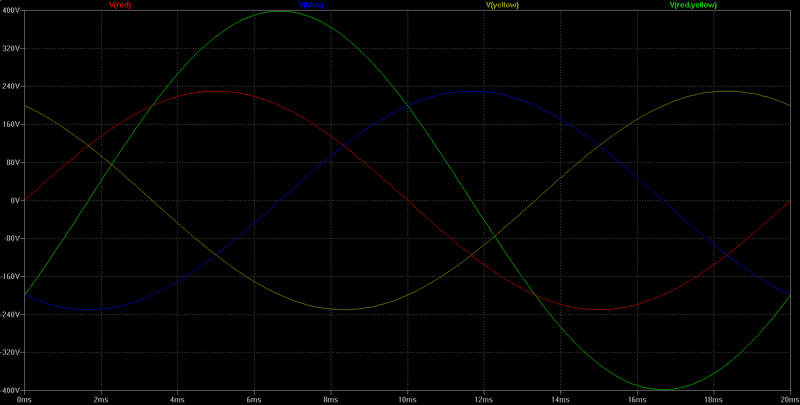# 3 Phase Systems in a Wye Configuration

Hello,

I have been going over the above topic and have a question about the neutral point of a balanced wye configuration. Would the line to line voltage remain constant? I am trying to picture two sine waves 120° apart and it's making me think the Va would be reducing as Vb was increasing at certain points? So would Vline still be alternating sinosoidally?

The reason I ask is I did an experiment in class where we had to set the line voltage to 10V and confirm it with a multimeter. Is it possible the multimeter was showing the peak value?

On another note, am I correct in thinking the voltage potential at the neutral point would be zero provided the system was balanced?

Thanks

Last edited:

## Answers and Replies

You are correct in your thinking that, if the system is balanced, the neutral potential would be zero.

You are also correct in your thinking that, as one of the phase to neutral voltages changes, the other two will also change such that Va + Vb + Vc = 0. The line to line voltages would also be sinusoidal.

You are correct in your thinking that, if the system is balanced, the neutral potential would be zero.

You are also correct in your thinking that, as one of the phase to neutral voltages changes, the other two will also change such that Va + Vb + Vc = 0. The line to line voltages would also be sinusoidal.

So that would mean the current Ia, Ib and Ic have a phase different with each other but at all times the sum of current at the neutral node would be 0?

Therefore in order for a system to be unbalanced the input voltage at A,B,C would either have to have a different peak or not be 120 degrees apart? Or have different loads?

Thanks

Baluncore
Science Advisor
So would Vline still be alternating sinosoidally?

Yes. The differential voltage between two phases is sinusoidal.

The line voltages will be symmetrical and separated by 120° about the neutral.

The current will be balanced if the load is balanced. The phase of the current will be determined by the impedance of the load.

If the load is not connected to the neutral then the neutral currents will cancel at the centre of the 'Y' and the neutral conductor will carry zero current.

See attached picture. It shows 3 phases, each 230Vpeak, NOT RMS.
It also shows one of the three, two phase differential line voltages, red – yellow.Yes. The differential voltage between two phases is sinusoidal.

The current will be balanced if the load is balanced. The phase of the current will be determined by the impedance of the load.

If the load is not connected to the neutral then the neutral currents will cancel at the centre of the 'Y' and the neutral conductor will carry zero current.

View attachment 203303

If the load at for example A wasn't connected then wouldn't that cause the system to become unbalanced as the the impedance at A would be infinite as it would be an open circuit?

Baluncore
Science Advisor
If the load at for example A wasn't connected then wouldn't that cause the system to become unbalanced as the the impedance at A would be infinite as it would be an open circuit?
The current will be balanced if the load is balanced.
If the load is unbalanced then the currents in the lines will be unbalanced.
If one line is disconnected, the total current flowing in the three lines will still sum to zero. There can be no neutral current.
The load may not function correctly if one phase is not connected.